# How To use Arithmetic operator using LINQ

Hello Everyone, Hope you are doing good

I would like to present this post who are looking for the expressions related to Arithmetic operator Min | Max | Sum | Average

# Introduction

Aggregate expressions are used to make calculations over a specific set of records. For example, to calculate the total Quantity of all records, or the total number of records in the dataset.

# Sum operator

``````SUM function can be extremely useful to calculate the sum of the items in a list/Collection
``````

### LINQ Expression

``````(From d in BuildDt.AsEnumerable Where Not (isNothing(d(1)) OrElse String.IsNullorEmpty(d(1).toString.Trim)) Select v = CDbl(d(1).toString.Trim)).Sum(Function (x) x)
``````
Output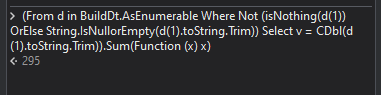# Average operator

``````The AVERAGE function can be used to find the average value of the items in a list/Collection.
``````

### LINQ Expression

``````(From d in BuildDt.AsEnumerable Where Not (isNothing(d(1)) OrElse String.IsNullorEmpty(d(1).toString.Trim)) Select v = CDbl(d(1).toString.Trim)).Average(Function (x) x)
``````
Output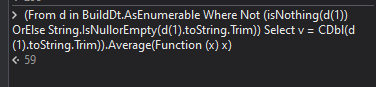# Minimum operator

``````MIN function can be used to find the minimum value in a list/Collection
``````

### LINQ Expression

``````(From d in BuildDt.AsEnumerable Where Not (isNothing(d(1)) OrElse String.IsNullorEmpty(d(1).toString.Trim)) Select v = CDbl(d(1).toString.Trim)).Min(Function (x) x)
``````
Output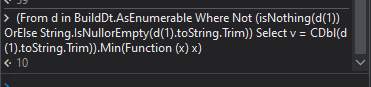# Maximum operator

``````MAX function can be used to find the maximum value in a list/Collection.
``````

### LINQ Expression

``````(From d in BuildDt.AsEnumerable Where Not (isNothing(d(1)) OrElse String.IsNullorEmpty(d(1).toString.Trim)) Select v = CDbl(d(1).toString.Trim)).Max(Function (x) x)
``````
Output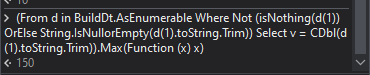You can find the sample workflow here

LINQ_ArithmeticOperator.xaml (9.3 KB)

Reference

Hope this will be helpful# Questions

For questions on your retrieval case open a new topic and get individual support

# Feedback

Click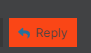button to share your thoughts

Regards
Gokul

7 Likes

@Gokul001 Nice

1 Like

I’m Appreciating you for Knowledge Sharing

1 Like